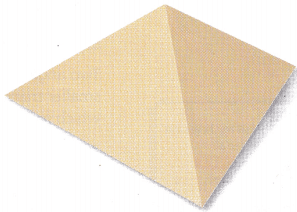# Texas Go Math Grade 8 Module 7 Answer Key Angle Relationships in Parallel Lines and Triangles

Refer to our Texas Go Math Grade 8 Answer Key Pdf to score good marks in the exams. Test yourself by practicing the problems from Texas Go Math Grade 8 Module 7 Answer Key Angle Relationships in Parallel Lines and Triangles.

## Texas Go Math Grade 8 Module 7 Answer Key Angle Relationships in Parallel Lines and Triangles

Solve for x.

Question 1.
6x + 10 = 46
Following the example on page 190, first we subtract 10 from both sides Then, we simplify and we divide both sides by 6.
6x + 10 = 46
6x + 10 – 10 = 46 – 10
6x = 36
$$\frac{6x}{6}$$ = $$\frac{36}{6}$$
x = 6

Question 2.
7x – 6 = 36
Following the example on page 190, first we add 6 to both sides Then, we simpLify and we divide both sides by 7.
7x – 6 = 36
7x – 6 + 6 = 36 + 6
7x = 42
$$\frac{7x}{7}$$ = $$\frac{42}{7}$$
x = 6

Question 3.
3x + 26 = 59
Following the example on page 190, first we subtract 26 from both sides. Then, we simplify and we divide both sides by3.
3x + 26 = 59
3x + 26 – 26 = 59 – 26
3x = 33
$$\frac{3x}{3}$$ = $$\frac{33}{3}$$
x = 11

Question 4.
2x + 5 = -25
Following the example on page 190, first we subtract 5 from both sides. Then, we simplify and we divide both sides by 2.
2x + 5 = 25
2x + 5 – 5 = -25 – 5
2x = 30
$$\frac{2x}{2}$$ = $$\frac{30}{2}$$
x = -15

Question 5.
6x – 7 = 41
6x – 7 = 41 (Write the equation) ……….. (1)
6x = 41 + 7 (Add 7 to both sides) ………… (2)
6x = 48 (Simplify) ………….. (3)
x = $$\frac{48}{6}$$ (Divide both sides by 6) ………. (4)
x = 8 (Simplify) ………….. (5)

Question 6.
$$\frac{1}{2}$$x + 9 = 30
$$\frac{1}{2}$$x + 9 = 30 (Write the equation.) ……………. (1)
$$\frac{1}{2}$$x = 30 – 9 (Subtract 9 from both sides) ……………. (2)
$$\frac{1}{2}$$x = 21 (Simplify) ………………. (3)
x = 21 ∙ 2 (Multiply both sides by 2) …………… (4)
x = 42 (Simplify) …………… (5)

Question 7.
$$\frac{1}{3}$$x – 7 = 15
$$\frac{1}{3}$$x + 7 = 15 (Write the equation.) ……………. (1)
$$\frac{1}{3}$$x = 15 + 7 (Add 7 from both sides) ……………. (2)
$$\frac{1}{3}$$x = 22 (Simplify) ………………. (3)
x = 22 ∙ 3 (Multiply both sides by 3) …………… (4)
x = 66 (Simplify) …………… (5)

Question 8.
0.5x – 0.6 = 8.4
0.5x – 0.6 = 8.4 (Write the equation) …………. (1)
0.5x = 8.4 + 0.6 (Subtract 9 from both sides) …………. (2)
0.5x = 9 (Simplify) ………….. (3)
x = $$\frac{9}{0.5}$$ (Divide both sides with 0.5) ………. (4)
x = 18 (Simplify) …………. (5)

Give two names for the angle formed by the dashed rays.

Question 9.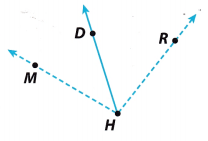The angle formed by the dashed rays can be named as: ∠MHR or ∠RHM

Question 10.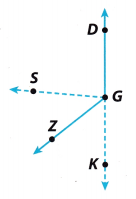The angle formed by the dashed rays can be named as: ∠SGK or ∠KGS

Question 11.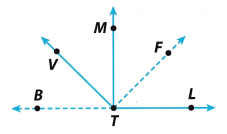The angle formed by the dashed rays can be named as: ∠BTF or ∠FTB

Visualize Vocabulary

Use the ✓ words to complete the graphic. You can put more than one word in each section of the triangle.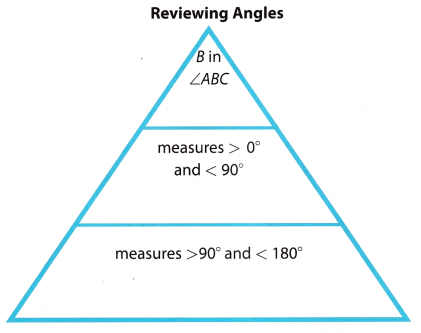Understand Vocabulary

Complete the sentences using preview words.

Question 1.
A line that intersects two or more lines is a ____________.
A transversal line is a line that intersects two or more Lines in the same plane at different points.
By definition, a line that intersects two or more lines is a transversal.
Thus, the blank part in the statement is transversal

Question 2.
Figures with the same shape but not necessarily the same size are ____________.
Figures with the same shape but not necessarily the same size are similar figures.

Question 3.
An ____________________ is an angle formed by one side of the triangle and the extension of an adjacent side.